# Grade - math word problems

#### Number of examples found: 5336

• Certificate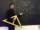There is 31 students in a class. From mathematics was'nt worse mark than 2. Average mark in mathematics was 2. How many students have mark 1 and how many mark 2?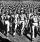Road repair took 10 days for 36 workers if they worked 9 hours a day. How many days take repair same road 37 workers if they work 8 per day?
• SavingMom said that Suzan save about 120 EUR (rounded to tens). How many euros she could at least save (minimum)?
• Building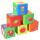Lenka has 22 cubes for the construction of building comprising three cubes in height, the width of two cubes, and the length of four cubes. Is she able to build an building with these cubes?
• Rhombus ABCDRhombus ABCD, |AC| = 90 cm, |BD| = 49 cm. Calculate the perimeter of the rhombus ABCD.
• DivisibilityIs the number 761082 exactly divisible by 9? (the result is the integer and/or remainder is zero)
• Tippler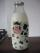Bottle with cork cost 8.8 Eur. The bottle is 0.8 euros more expensive than cork. How much is a bottle and the cork?
• A bottleA bottle full of cola weighs 1,320 g. If we drink three-tenths of it, it will weigh 1,008g. How much does an empty bottle weigh?
• The tourist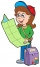The tourist walked a quarter of the way on the first day, a third of the rest on the second day, and 20 km on the last day. How many km did he walked in three days?
• Diamond area from diagonalsIn the diamond ABCD is AB = 4 dm and the length of the diagonal is 6.4 dm long. What is the area of the diamond?
• Cylinder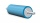The 1.8m cylinder contains 2000 liters of water. What area (in dm2) of this container is the water?
• Hexagon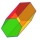Calculate the surface of a regular hexagonal prism whose base edge a = 12cm and side edge b = 3 dm.
• QuotientFind quotient before the bracket - the largest divisor 51 a + 34 b + 68 121y-99z-33
• In centerWhat number lies in the center between a quarter of a fifth and a half of a third on the number axis?
• Diamond and diagonalsA diamond has diagonals f = 8 cm and g = 6 cm long. How long is this diamond perimeter? (Calculate it!)
• Poplar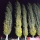How tall is a poplar by the river, if we know that 1/5 of its total height is a trunk, 1/10th of the height is the root and 35m from the trunk to the top of the poplar?
• The sizeThe size of a Trapezium are 3/4×cm, ×cm 2(×+1)cm and 3(×+2)cm long respectively if it's perimeter is 60cm, calculate the length of each side.
• Jose and Kaitlyn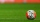Jose and Kaitlyn have a contest to see who can throw a baseball the farthest. Kaitlyn wins, with a throw of 200 ft. If Jose threw the ball 3/4 as far as Kaitlyn, how far did Jose throw the ball?
• Triplets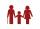Three years ago, the triplets Pavel, Petr, and Luke and their five-year-old sister Jane had together 25 years. How old is Jane today?
• BasenHow many square meters of tiles we need to tile the walls and floor of the pool 15 meters long, six meters wide and two meters?deep

Do you have an interesting mathematical word problem that you can't solve it? Submit a math problem, and we can try to solve it.

We will send a solution to your e-mail address. Solved examples are also published here. Please enter the e-mail correctly and check whether you don't have a full mailbox.

Please do not submit problems from current active competitions such as Mathematical Olympiad, correspondence seminars etc...